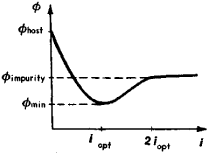# work function

(redirected from Work function (thermodynamics))
Also found in: Dictionary.

## Work function (electronics)

A quantity with the dimensions of energy which determines the thermionic emission of a solid at a given temperature. For metals, the work function may also be determined by measuring the photoemission as a function of the frequency of the incident electromagnetic radiation: the work function is then equal to the minimum (threshold) frequency for which electon emission is observed times Planck's constant h (= 6.63 × 10-34 joule second). The work function of a solid is usually expressed in electronvolts.

The work function of metals varies from one crystal plane to another and also varies slightly with temperature. For a metal, the work function has a simple interpretation. At absolute zero, the energy of the most energetic electrons in a metal is referred to as the Fermi energy; the work function of a metal is then equal to the energy required to raise an electron with the Fermi energy to the energy level corresponding to an electron at rest in vacuum. The work function of a semiconductor or an insulator has the same interpretation, but in these materials the Fermi level is in general not occupied by electrons and thus has a more abstract meaning. See Field emission, Photoemission, Thermionic emission

## Work function (thermodynamics)

The thermodynamic function better known as the Helmholtz energy, A = U - TS, where U is the internal energy, T is the thermodynamic (absolute) temperature, and S is the entropy of the system. At constant temperature, the change in work function is equal to the maximum work that can be done by a system (ΔA = wmax). See Free energy

## Work Function

the energy expended in the removal of an electron from a solid or liquid into a vacuum. The passage of an electron from a vacuum to a condensed medium is accompanied by the release of an amount of energy equal to the work function Φ = ep (e is the charge of an electron, ρ is the work function potential). Consequently, the work function is a measure of the bond between the electron and the condensed medium; the smaller the work function, the more readily will electron emission occur. The current density of, for example, thermionic emission or autoelectronic emission is therefore exponentially related to the work function.

The work function has been most thoroughly studied for conductors, especially metals, where the function depends on the crystallographic orientation of the surface. The more densely the crystal face is “packed,” the larger is the work function. For example, for pure tungsten, Φ = 4.3 electron volts (eV) for {116} faces and 5.35 eV for {110} faces. For metals, the increase in Φ (averaged for the faces) corresponds approximately to the increase in the ionization potential. The smallest work functions (2 eV) belong to the alkaline-earth metals (Cs, Rb, K), while the largest (5.5 eV) belong to the metals of the Pt group.

The work function is sensitive to defects in the surface structure. Atoms of the material on a densely packed face that are disorderly positioned reduce Φ. There is an even stronger dependence of Φ on surface impurities. Electronegative impurities (oxygen, halogens, metals with a Φ greater than that of the substrate) usually increase Φ, while electropositive impurities have the opposite effect. For most electropositive impurities (Cs in W, Th in W, Ba in W), a decrease in the work function is observed; with an optimum concentration of impurities iopt the function will reach a minimum value, which is lower than the Φ of the base metal. When i ≈ 2iopt, the work function approximates the Φ of the impurity metal and does not then undergo further change (Figure 1). An ordered layer of impurity atoms fitted to the structure of the substrate and, as a rule, filling all vacancies corresponds to the value of iopt. A dense monatomic layer not fitted to the structure of the substrate corresponds to the value of 2iopt. Thus, at least for materials exhibiting metallic electrical conductivity, the work function is determined by the surface properties.Figure 1. Dependence of the work function ɸ on the surface concentration i of electropositive impurity atoms

The electron theory of metals regards the work function as the work necessary for the removal of an electron from the Fermi level into a vacuum. Current theory does not yet permit a precise determination of Φ for specific structures and surfaces. Most information on the values of Φ is derived experimentally. Values can be determined by using emission or contact phenomena.

Knowledge of the work function is essential in designing vacuum-tube devices that use the emission of electrons or ions and such devices as thermionic converters.

### REFERENCES

Dobretsov, L. N., and M. V. Gomoiunova. Emissionnaia elektronika. Moscow, 1966.
Zandberg, E. Ia., and N. I. Ionov. Poverkhnostnaia ionizatsiia. Moscow, 1969.

V. N. SHREDNTK

## work function

[′wərk ‚fəŋk·shən]
(solid-state physics)
The minimum energy needed to remove an electron from the Fermi level of a metal to infinity; usually expressed in electronvolts.
(thermodynamics)

## work function

The amount of photon energy required to cause an electron to be emitted from a material.
Site: Follow: Share:
Open / Close